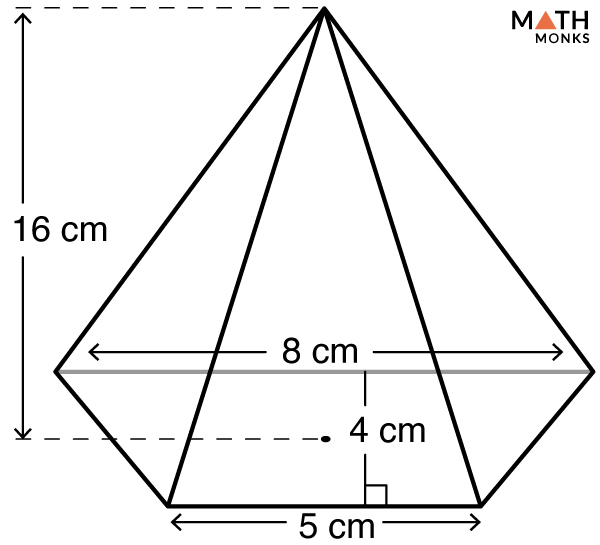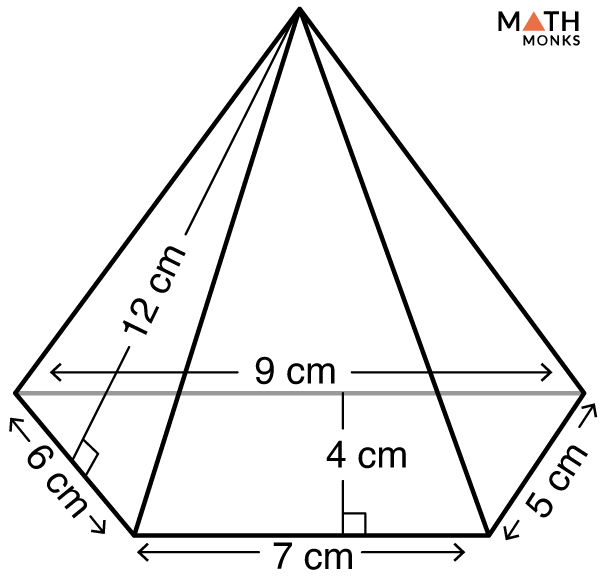# Trapezoidal Pyramid

A trapezoidal pyramid is a pyramid with a trapezoidal base bounded by four lateral faces meeting at a common point, known as the apex. The lateral faces are in the shape of triangles.

How many faces, edges and vertices does a trapezoidal pyramid have?

A trapezoidal pyramid has 5 faces, 8 edges, and 5 vertices.

## Formulas

### Volume

The formula is:

Volume (V) = ${\dfrac{1}{6}\times h\left( b_{1}+b_{2}\right) \times H}$, here b1 & b2 = two parallel side lengths of the trapezoidal base, h = base height, H = height of pyramid

Let us solve an example to understand the concept better.Find the volume of a trapezoidal pyramid given in the figure.

Solution:

As we know,
Volume (V) = ${\dfrac{1}{6}\times h\left( b_{1}+b_{2}\right) \times H}$, here b1 = 5 cm, b2 = 8 cm, h = 4 cm, H = 16 cm
V = ${\dfrac{1}{6}\times 4\times \left( 5+8\right) \times 16}$
= 138.67 cm3

### Surface Area

The formula is:

Surface Area (SA) = ${B+\dfrac{1}{2}\times P\times s}$, here B = Base Area, P = Base Perimeter, s = slant height

As we know,

Base Area (B) = ${\dfrac{1}{2}\left( a+b\right) \times h}$, here a & b = 2 parallel base side lengths, h = base height (area of a trapezoid)

½ (a + b) × h, here a & b = 2 parallel base side lengths, h = base height (area of a trapezoid)

Base Perimeter (P) = a + b + c + d, here a, b, c, & d = base side lengths (perimeter of a trapezoid)

Let us solve an example to understand the concept better.Find the surface area of a trapezoidal pyramid given in the figure.

Solution:

As we know,
Surface Area (SA) = ${B+\dfrac{1}{2}Ps}$
Solving for B & P,
B = ${\dfrac{1}{2}\left(a+b\right) \times h}$, here a = 9 cm, b = 7 cm, h = 4 cm
∴ B = ${\dfrac{1}{2}\times \left(9+7\right) \times 4}$
= 32 cm2
Base Perimeter (P) = a + b + c + d, here a = 9 cm, b = 7 cm, c = 6 cm, d = 5 cm
∴ P = 9 + 7 + 6 + 5
= 27 cm
Now, Solving for SA
SA = ${B+\dfrac{1}{2}Ps}$, here B = 32 cm2 , P = 27 cm, s = 12 cm
∴ SA = ${32+\dfrac{1}{2}\times 27\times 12}$
= 194 cm2